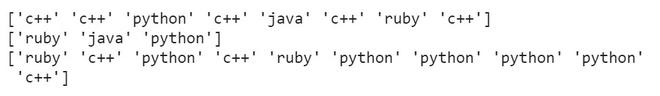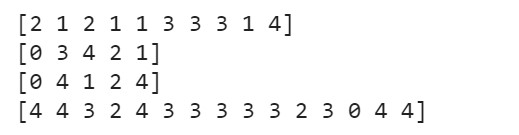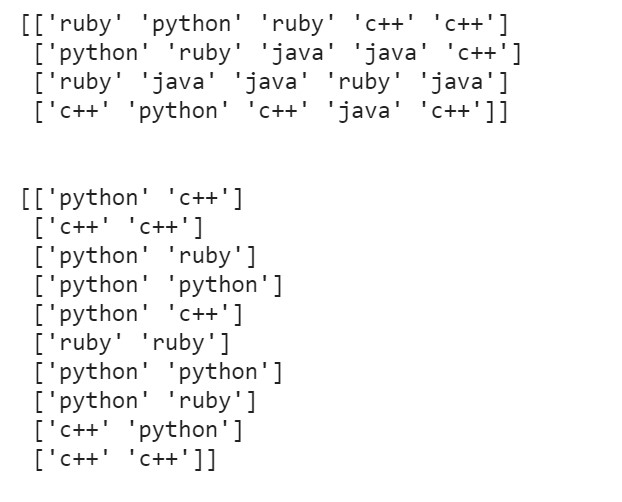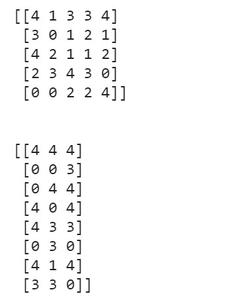Related Articles

# How to randomly select elements of an array with NumPy in Python ?

• Last Updated : 15 Mar, 2021

Prerequisites: Numpy

The random values are useful in data-related fields like machine learning, statistics and probability. The numpy.random.choice() function is used to get random elements from a NumPy array. It is a built-in function in the NumPy package of python.

Syntax: numpy.random.choice( a , size = None, replace = True, p = None)

Parameters:

• a: a one-dimensional array/list (random sample will be generated from its elements) or an integer (random samples will be generated in the range of this integer)
• size: int or tuple of ints (default is None where a single random value is returned). If the given shape is (m,n), then m x n  random samples are drawn.
• replace: (optional); the Boolean value that specifies whether the sample is drawn with or without replacement. When sample is larger than the population of the list, replace cannot be False.
• p: (optional); a 1-D array containing probabilities associated with each entry in a. If not given then sample assumes uniform distribution over all entries in a.

### Approach

• Import module
• Create a sample array
• Randomly choose values from the array created
• Print the array so generated.

Given below is the implementation for 1D and 2D array.

Generating 1-D list of random samples

Example 1:

## Python3

 `import` `numpy as np`` ` `prog_langs ``=` `[``'python'``, ``'c++'``, ``'java'``, ``'ruby'``]`` ` `# generating random samples``print``(np.random.choice(prog_langs, size``=``8``))`` ` `# generating random samples without replacement``print``(np.random.choice(prog_langs, size``=``3``, replace``=``False``))`` ` `# generating random samples with probabilities``print``(np.random.choice(prog_langs, size``=``10``,``                       ``replace``=``True``, p``=``[``0.3``, ``0.5``, ``0.0``, ``0.2``]))`

Output :Example 2:

## Python3

 `import` `numpy as np`` ` `samples ``=` `5``# generating random samples``print``(np.random.choice(samples, size``=``10``))`` ` `# generating random samples without replacement``print``(np.random.choice(samples, size``=``5``, replace``=``False``))`` ` `# generating random samples with probablities``print``(np.random.choice(samples, size``=``5``, replace``=``True``))`` ` `# generating with probabilities``print``(np.random.choice(samples, size``=``15``,``                       ``replace``=``True``, p``=``[``0.2``, ``0.1``, ``0.1``, ``0.3``, ``0.3``]))`

Output:Generating a 2-D list of random samples

Example:

## Python3

 `import` `numpy as np`` ` `prog_langs ``=` `[``'python'``, ``'c++'``, ``'java'``, ``'ruby'``]`` ` `# generating random samples``print``(np.random.choice(prog_langs, size``=``(``4``, ``5``)))`` ` `# generating random samples with probabilities``print``(``'\n'``)``print``(np.random.choice(prog_langs, size``=``(``10``, ``2``),``                       ``replace``=``True``, p``=``[``0.3``, ``0.5``, ``0.0``, ``0.2``]))`

Output:Example 2:

## Python3

 `import` `numpy as np`` ` `samples ``=` `5`` ` `# generating random samples``print``(np.random.choice(samples, size``=``(``5``, ``5``)))`` ` `# generating with probabilities``print``(``'\n'``)``print``(np.random.choice(samples, size``=``(``8``, ``3``),``                       ``replace``=``True``,``                       ``p``=``[``0.2``, ``0.1``, ``0.1``, ``0.3``, ``0.3``]))`

Output:Attention geek! Strengthen your foundations with the Python Programming Foundation Course and learn the basics.

To begin with, your interview preparations Enhance your Data Structures concepts with the Python DS Course. And to begin with your Machine Learning Journey, join the Machine Learning – Basic Level Course

My Personal Notes arrow_drop_up